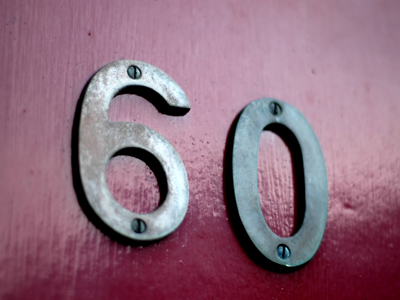6 x 10 = 60.

# The 10 Times Table

The ten times table may well be the easiest of them all - but you still need to learn it. It'll come in very useful in everyday life and in KS3 Maths. So, even though you may think it's very simple, practise it all the same.

Multiplication by 10 is what the ten times table is all about. Ten is a very important number because the metric system bases its units on multiples of ten. There are tem millimetres in a centimetre, a hundred centimetres in a metre, a thousand metres in a kilometre - the list goes on. Grams, litres, hectares. They all work by using multiples of ten. Thankfully there is a very simple way to multiply a number by ten - you just add a zero to its end. 7 x 10 = 70. 56 x 10 = 560. 1,267 x 10 = 12,670. What could be easier?

With that in mind, you may be feeling very confident of getting 10 out of 10 in this quiz - but be careful. If you don't concentrate then you might click on the wrong answer by mistake. Take your time and consider your answer before you make your choice. Good luck!

1.
What is 1 x 10
1000
10
100
10000
Any number multiplied by one remains unchanged
2.
What is 9 x 10
99
60
30
90
90 is 10 less than 100, which is 10 x 10
3.
What is 7 x 10
80
70
50
60
Add a zero after the 7 to multiply it by 10
4.
What is 4 x 10
40
30
20
50
4 x 10 is the same as 10 + 10 + 10 + 10
5.
What is 6 x 10
80
90
30
60
There are 60 seconds in a minute and 60 minutes in an hour
6.
What is 5 x 10
25
50
60
100
50 is the same as one half of 10 x 10
7.
What is 10 x 10
20
100
50
80
10 is the square root of 100
8.
What is 2 x 10
20
30
40
50
There are 20 sectors on a dartboard
9.
What is 8 x 10
90
40
80
88
Just add a 0 after the 8 and you get the answer 80
10.
What is 3 x 10
60
35
90
30
10 x 3 is found by adding a 0 to the end of 3
Author:  Frank Evans